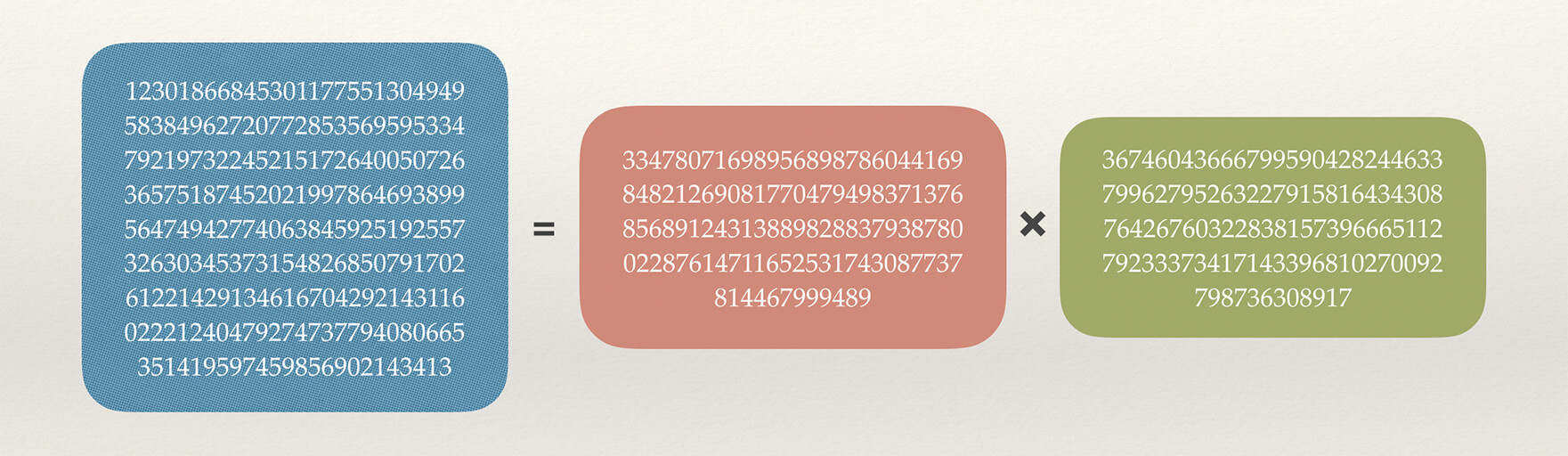• RSA在公钥和密文传输的过程中就真的很安全吗？
• 为什么解密的时候原文：m 就等于 $c^d$ % n呢？
• m<n，这就导致RSA加密的内容长度受到了限制，那如何加密发送一段很长的文本呢？

## 简单回顾

### 1.生成共钥、私钥

1. 找出质数p、q，p≠q.
2. 计算n = p x q.
3. 计算n的欧拉函数φ(n)=(p-1)(q-1)
4. 选取整数e，条件是1< e < φ(n)，且e与φ(n) 互质，则公钥为:（e,n)
5. 计算e对于φ(n)的模反元素d，将d和当作私钥:（d,n)

### 2.加密、解密

1. 加密：用公钥对信息m加密，计算密文：c = $m^e​$ % n.
2. 解密：用私钥对密文c解密，计算原文：m = $c^d$ % n.

## 安全性

ed % φ(n) = 1

e在公钥中我们已知，要求d，所以求出φ(n)就行，那φ(n)又怎么求呢？通过上面生成共私钥步骤3：

φ(n)=(p-1)(q-1)

n = p x q

n在公钥中我们已知，只需要分解n就可以，但在实际应用中，n一般取1024位或更大的2048位，在第一篇文章中我们知道分解大数的质因数是当今三大数学难题之一，所以RSA加密在理论上是安全的。当今人类被报道破解的最大密钥长度是768位，232个十进制数，写下来如下:## 解密原理

$c^d$ % n = m

1. 根据加密公式，可转换为c = $m^e-kn$.
2. 将上式带入解密公式 m = $c^d$ % n 得到： $(m^e-kn)^d$ % n = m.
3. 因为$(m^e-kn)^d$ 中第二项含有kn是n的倍数，所以不管d是多少，乘开后，只有$m^{ed}$项不含有n，而含有n的项都能被n整除，所以上式可以简化成 $m^{ed}$ % n = m.
4. 上面加密过程第5步求模反元素公式： ed % φ(n) = 1，可写成 ed = hφ(n)+1，将其带入$m^{ed}$ % n = m

• m、n互质
1. 由欧拉定理可知 $m^{φ(n)}$%n = 1.
2. 上式可变为：$(m^{φ(n)})^h$ % n = 1，例如$5^1$%4=1、$5^2$%4=1、$5^3$%4=1…
3. $(m^{φ(n)})^h$ % n = 1 等价于 $m^{hφ(n)}$ % n = 1，可写成$m·m^{hφ(n)}$ % n = m，例如5%4=1、2·5%4=2、3·5%4=3、4·5%4=4
4. $m·m^{hφ(n)}$ % n = m 可写成 $m^{hφ(n)+1}$ % n = m，原式得证！
• m、n不互质
1. 由加密过程：n = p x q，故n只能被分解成p和q的乘积，因为m和n不互质，故m一定是p或q的倍数，以m=kp为例，此时kp和q互质。
2. 根据欧拉定理有：$(kp)^{φ(q)}$ % q = 1即：$(kp)^{q-1}$ % q = 1.
3. 上式进行h(p-1)次方运算再乘kp后得到：$[ (kp)^{q-1}]^{h(p-1)}$ x kp % q = kp，左边合并指数后得到：$(kp)^{h(p-1)(q-1)+1}$ % q = kp.
4. 从上面我们已知 ed = hφ(n)+1即ed =h(p-1)(q-1)+1，所以上式可简化成：$(kp)^{ed}$ % q = kp.
5. 上式可写成$(kp)^{ed}$ = tq + kp，可以发现，等式左边肯定是p的倍数，右边kp是p的倍数，所以tq也是p的倍数，而p和q互质，所以t一定能被p整除，即一定有 t =t’p，带入可得：$(kp)^{ed}$ = t’pq + kp.
6. 从上面可知m=kp、n=pq，所以带入上式得: $m^{ed}$ = t’n + m，即：$m^{ed}$ % n = m，原式得证！

## 加密长度限制

1. 分段加密：即可以通过将发送的消息分成多段，分别加密，再进行传输。
2. 结合AES加密：可通过对称性加密算法AES对长文本进行快速加密，再通过RSA加密算法对AES加密的密钥进行加密。